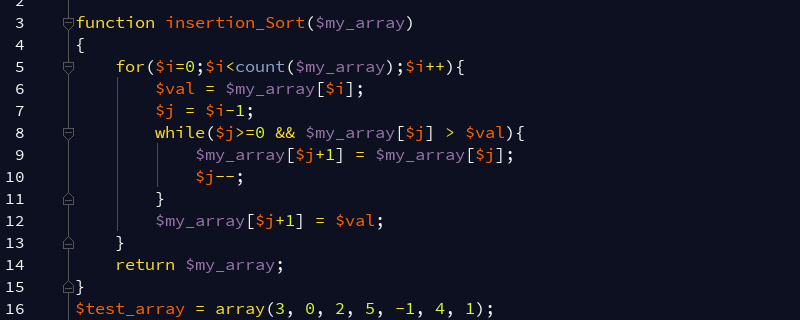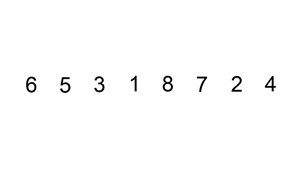# PHP如何实现插入排序？PHP插入排序的代码示例如下：

```<?php

function insertion_Sort(\$my_array)
{
for(\$i=0;\$i<count(\$my_array);\$i++){
\$val = \$my_array[\$i];
\$j = \$i-1;
while(\$j>=0 && \$my_array[\$j] > \$val){
\$my_array[\$j+1] = \$my_array[\$j];
\$j--;
}
\$my_array[\$j+1] = \$val;
}
return \$my_array;
}
\$test_array = array(3, 0, 2, 5, -1, 4, 1);
echo "原始数组:\n";
echo implode(', ',\$test_array );
echo "\n排序后数组 :\n";
print_r(insertion_Sort(\$test_array));```

```原始数组: 3, 0, 2, 5, -1, 4, 1

Array (
 => -1
 => 0
 => 1
 => 2
 => 3
 => 4
 => 5
)```